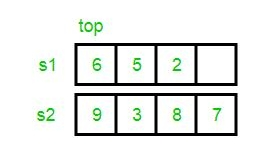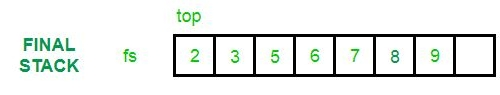# Merging and Sorting Two Unsorted Stacks

• Difficulty Level : Medium
• Last Updated : 14 Jun, 2022

Given 2 input stacks with elements in an unsorted manner. Problem is to merge them into a new final stack, such that the elements become arranged in a sorted manner.Examples:

```Input :  s1 : 9 4 2 1
s2: 8 17 3 10
Output : final stack: 1 2 3 4 8 9 10 17

Input :  s1 : 5 7 2 6 4
s2 : 12 9 3
Output : final stack: 2 3 4 5 6 7 9 12```

Create an empty stack to store result. We first insert elements of both stacks into the result. Then we sort the result stack.

## C++

 `// C++ program to merge to unsorted stacks``// into a third stack in sorted way.``#include ``using` `namespace` `std;` `// Sorts input stack and returns sorted stack.``stack<``int``> sortStack(stack<``int``>& input)``{``    ``stack<``int``> tmpStack;` `    ``while` `(!input.empty()) {``        ``// pop out the first element``        ``int` `tmp = input.top();``        ``input.pop();` `        ``// while temporary stack is not empty and top``        ``// of stack is greater than temp``        ``while` `(!tmpStack.empty() && tmpStack.top() > tmp) {` `            ``// pop from temporary stack and push``            ``// it to the input stack``            ``input.push(tmpStack.top());``            ``tmpStack.pop();``        ``}` `        ``// push temp in temporary of stack``        ``tmpStack.push(tmp);``    ``}` `    ``return` `tmpStack;``}` `stack<``int``> sortedMerge(stack<``int``>& s1, stack<``int``>& s2)``{``    ``// Push contents of both stacks in result``    ``stack<``int``> res;``    ``while` `(!s1.empty()) {``        ``res.push(s1.top());``        ``s1.pop();``    ``}``    ``while` `(!s2.empty()) {``        ``res.push(s2.top());``        ``s2.pop();``    ``}` `    ``// Sort the result stack.``    ``return` `sortStack(res);``}` `// main function``int` `main()``{``    ``stack<``int``> s1, s2;``    ``s1.push(34);``    ``s1.push(3);``    ``s1.push(31);` `    ``s2.push(1);``    ``s2.push(12);``    ``s2.push(23);` `    ``// This is the temporary stack``    ``stack<``int``> tmpStack = sortedMerge(s1, s2);``    ``cout << ``"Sorted and merged stack :\n"``;` `    ``while` `(!tmpStack.empty()) {``        ``cout << tmpStack.top() << ``" "``;``        ``tmpStack.pop();``    ``}``}`

## Java

 `// Java program to merge to unsorted stacks``// into a third stack in sorted way.``import` `java.io.*;``import` `java.util.*;` `public` `class` `GFG {``    ` `    ``// This is the temporary stack``    ``static` `Stack res = ``new` `Stack();``    ``static` `Stack tmpStack = ``new` `Stack();``    ` `    ``// Sorts input stack and returns``    ``// sorted stack.``    ``static` `void` `sortStack(Stack input)``    ``{``        ``while` `(input.size() != ``0``)``        ``{``            ``// pop out the first element``            ``int` `tmp = input.peek();``            ``input.pop();``    ` `            ``// while temporary stack is not empty and``            ``// top of stack is greater than temp``            ``while` `(tmpStack.size() != ``0` `&&``                            ``tmpStack.peek() > tmp)``            ``{``    ` `                ``// pop from temporary stack and push``                ``// it to the input stack``                ``input.push(tmpStack.peek());``                ``tmpStack.pop();``            ``}``    ` `            ``// push temp in temporary of stack``            ``tmpStack.push(tmp);``        ``}``    ``}``    ` `    ``static` `void` `sortedMerge(Stack s1,``                                ``Stack s2)``    ``{``        ``// Push contents of both stacks in result``        ``while` `(s1.size() != ``0``) {``            ``res.push(s1.peek());``            ``s1.pop();``        ``}``        ` `        ``while` `(s2.size() != ``0``) {``            ``res.push(s2.peek());``            ``s2.pop();``        ``}``    ` `        ``// Sort the result stack.``        ``sortStack(res);``    ``}``    ` `    ``// main function``    ``public` `static` `void` `main(String args[])``    ``{``        ``Stack s1 = ``new` `Stack();``        ``Stack s2 = ``new` `Stack();``        ``s1.push(``34``);``        ``s1.push(``3``);``        ``s1.push(``31``);``    ` `        ``s2.push(``1``);``        ``s2.push(``12``);``        ``s2.push(``23``);``    ` `        ``sortedMerge(s1, s2);``        ``System.out.println(``"Sorted and merged stack :"``);``    ` `        ``while` `(tmpStack.size() != ``0``) {``            ``System.out.print(tmpStack.peek() + ``" "``);``            ``tmpStack.pop();``        ``}``    ``}``}` `// This code is contributed by Manish Shaw``// (manishshaw1)`

## Python3

 `# Python3 program to merge to unsorted stacks``# into a third stack in sorted way.` `# Sorts input stack and returns``# sorted stack.``def` `sortStack(``Input``):``    ``tmpStack ``=` `[]``  ` `    ``while` `len``(``Input``) !``=` `0``:``        ``# pop out the first element``        ``tmp ``=` `Input``[``-``1``]``        ``Input``.pop()``  ` `        ``# while temporary stack is not empty and``        ``# top of stack is greater than temp``        ``while` `len``(tmpStack) !``=` `0` `and` `tmpStack[``-``1``] > tmp:``            ``# pop from temporary stack and push``            ``# it to the input stack``            ``Input``.append(tmpStack[``-``1``])``            ``tmpStack.pop()``        ``# push temp in temporary of stack``        ``tmpStack.append(tmp)``    ``return` `tmpStack``  ` `def` `sortedMerge(s1, s2):``    ``# Push contents of both stacks in result``    ``res ``=` `[]``    ``while` `len``(s1) !``=``0` `:``        ``res.append(s1[``-``1``])``        ``s1.pop()``    ``while` `len``(s2) !``=``0` `:``        ``res.append(s2[``-``1``])``        ``s2.pop()``  ` `    ``# Sort the result stack.``    ``return` `sortStack(res)` `s1 ``=` `[]``s2 ``=` `[]``s1.append(``34``)``s1.append(``3``)``s1.append(``31``)` `s2.append(``1``)``s2.append(``12``)``s2.append(``23``)` `# This is the temporary stack``tmpStack ``=` `[]``tmpStack ``=` `sortedMerge(s1, s2)``print``(``"Sorted and merged stack :"``)` `while` `len``(tmpStack) !``=` `0` `:``    ``print``(tmpStack[``-``1``], end ``=` `" "``)``    ``tmpStack.pop()``    ` `    ``# This code is contributed by decode2207.`

## C#

 `// C# program to merge to unsorted stacks``// into a third stack in sorted way.``using` `System;``using` `System.Collections.Generic;` `class` `GFG {``    ` `    ``// Sorts input stack and returns``    ``// sorted stack.``    ``static` `Stack<``int``> sortStack(``ref` `Stack<``int``> input)``    ``{``        ``Stack<``int``> tmpStack = ``new` `Stack<``int``>();``    ` `        ``while` `(input.Count != 0)``        ``{``            ``// pop out the first element``            ``int` `tmp = input.Peek();``            ``input.Pop();``    ` `            ``// while temporary stack is not empty and``            ``// top of stack is greater than temp``            ``while` `(tmpStack.Count != 0 &&``                              ``tmpStack.Peek() > tmp)``            ``{``    ` `                ``// pop from temporary stack and push``                ``// it to the input stack``                ``input.Push(tmpStack.Peek());``                ``tmpStack.Pop();``            ``}``    ` `            ``// push temp in temporary of stack``            ``tmpStack.Push(tmp);``        ``}``    ` `        ``return` `tmpStack;``    ``}``    ` `    ``static` `Stack<``int``> sortedMerge(``ref` `Stack<``int``> s1,``                                  ``ref` `Stack<``int``> s2)``    ``{``        ``// Push contents of both stacks in result``        ``Stack<``int``> res = ``new` `Stack<``int``>();``        ``while` `(s1.Count!=0) {``            ``res.Push(s1.Peek());``            ``s1.Pop();``        ``}``        ``while` `(s2.Count!=0) {``            ``res.Push(s2.Peek());``            ``s2.Pop();``        ``}``    ` `        ``// Sort the result stack.``        ``return` `sortStack(``ref` `res);``    ``}``    ` `    ``// main function``    ``static` `void` `Main()``    ``{``        ``Stack<``int``> s1 = ``new` `Stack<``int``>();``        ``Stack<``int``> s2 = ``new` `Stack<``int``>();``        ``s1.Push(34);``        ``s1.Push(3);``        ``s1.Push(31);``    ` `        ``s2.Push(1);``        ``s2.Push(12);``        ``s2.Push(23);``    ` `        ``// This is the temporary stack``        ``Stack<``int``> tmpStack = ``new` `Stack<``int``>();``        ``tmpStack = sortedMerge(``ref` `s1,``ref` `s2);``        ``Console.Write(``"Sorted and merged stack :\n"``);``    ` `        ``while` `(tmpStack.Count!=0) {``            ``Console.Write(tmpStack.Peek() + ``" "``);``            ``tmpStack.Pop();``        ``}``    ``}``}` `// This code is contributed by Manish Shaw``// (manishshaw1)`

## Javascript

 ``

Output:

```Sorted and merged stack :
34 31 23 12 3 1```

Time Complexity: O((n+m)2) where n and m are number of elements in both the stacks respectively.
Auxiliary Space: O(n+m)

My Personal Notes arrow_drop_up